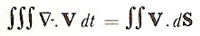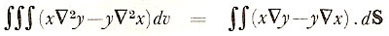A# Green's theorem

In vector analysis, Green's theorem is an extension of the divergence theorem, which latter states:where V is a vector and dS an element of a surface. If V has components (x, y, z) for a volume v and a surface S, Green's theorem states:Green's theorem provides a connection between path integrals over a well-connected region in the plane and the area of the region bounded in the plane. It is a form of the fundamental theorem of calculus, and is used today in almost all computer codes that solve partial differential equations.